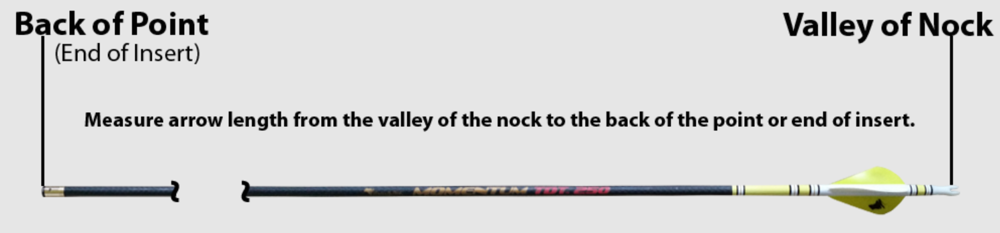# GrizzlyStik FOC Calculator

The AMO-Standard F.O.C. balance formula is: %FOC = (100x(A-L/2))/L

For those of you who prefer to calculate your FOC the old way with a calculator, this is for you:

A = Balance point as measured from the throat of the nock to the point of balance.
L = Total arrow length as measured from the throat of the nock to the back of the point.
(Do not include the length of the point in this measurement.)

1. Measure the arrow length from the throat of the nock to the end of the shaft. (Back of the point) e.g. 28.5"

2. Divide the total length number by two (2). (Or multiply it by 0.5.) e.g. 28.5/2 = 14.25 or 28.5 x 0.5 = 14.25

3. Find the arrow's balance point. Measured from the nock throat to the balance point. e.g. 19.0"

4. Subtract half the total length from the balance point number. e.g. 19.0" - 14.25" = 4.75"

5. Multiply that result by 100. e.g. 4.75 x 100 = 475

6. Now divide that number by the total arrow length. e.g. 475 / 28.5 = 16.6% FOC

Example:
A 28.50 (BOP) arrow has a balance point of 19.00

Half of the total arrow length is 14.25.

Subtract 14.25 from the 19" and you get 4.75".

Multiply that by 100 = 475.

Now divide 475 by the total length which is 28.5 = 16.6.